# Samacheer Kalvi 7th Maths Solutions Term 3 Chapter 5 Statistics Intext Questions

Students can Download Maths Chapter 5 Statistics Intext Questions and Answers, Notes Pdf, Samacheer Kalvi 7th Maths Book Solutions Guide Pdf helps you to revise the complete Tamilnadu State Board New Syllabus and score more marks in your examinations.

## Tamilnadu Samacheer Kalvi 7th Maths Solutions Term 3 Chapter 5 Statistics Intext Questions

Exercise 5.1
Try These (Text book Page No. 96)

Question 1.
Collect the height of students of your class. Organise the data in ascending order.
Solution:
Height of 15 students in our class.
130cm, 150 cm, 155 cm, 142 cm, 138 cm, 145 cm, 148 cm, 147 cm, 148cm, 143 cm, 141cm, 152 cm, 147 cm, 139 cm, 155 cm.

Ascending order:
130cm, 138cm, 139cm, 141cm, 142cm, 143cm, 145cm, 147cm, 147cm, 148cm, 148 cm, 150cm, 152 cm, 155cm, 155cm.Try These (Text book Page No. 97)

Find the Arithmetic Mean or average of the following data.

Question 1.
The study time spent by Kathir in a week is 3 hrs, 4 hrs, 5 hrs, 3 hrs, 4 hrs, 3:45 hrs; 4:15 hrs.
Solution: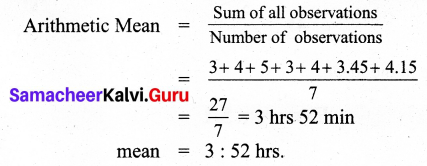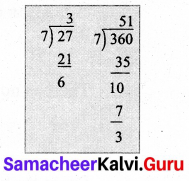mean = 3 : 52 hrs

Question 2.
The marks scored by Muhil in five subjects are 75, 91, 48, 63, 51.
Solution: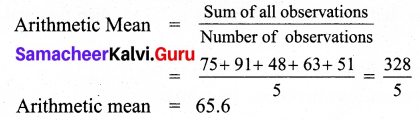Arithmetic Mean = 65.6Question 3.
Money spent on vegetables for five days is ₹ 120, ₹ 80, ₹ 75, ₹ 95 and ₹ 86.
Solution: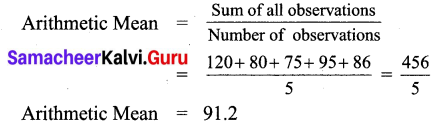Arithmetic Mean = 91.2

Think (Text book Page No. 99)

Check the properties of arithmetic mean for the example given below: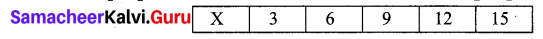Question 1.
If the mean is increased by 2, then what happens to the individual observations.
Solution:
Given number are 3, 6, 9, 12, 15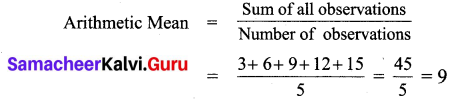If mean is increased by 2 then,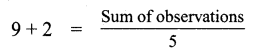Sum of observations = 5 × 11 = 55
Difference in sum = 55 – 45 = 10
∴ Each number is increased by 2 if the mean is increased by 2.

Question 2.
If first two items are increased by 3 and last two items are reduced by 3, then what will be the new mean?
Solution:
If the first two items is increased by 3, then the numbers will be 3 + 3, 6 + 3 ⇒ 6, 9.
If last two numbers are decreased by 3, then the numbers will be 12 – 3, 15 – 3 ⇒ 9,12.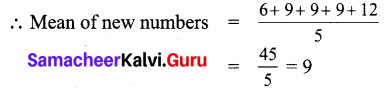= $$\frac { 45 }{ 5 }$$ = 9
There is no change in the mean.Exercise 5.2
Try These (Text book Page No. 101)

Question 1.
Find the mode of the following data. 2, 6, 5, 3, 0, 3, 4, 3, 2, 4, 5, 2
Solution:
Arranging the numbers in ascending order we get 0, 2, 2, 2, 3, 3, 3, 4, 4, 5, 5, 6
Since 2 and 3 occurs the maximum of 3 times. So mode of this data is 2 and 3.

Question 2.
Find the mode of the following data set. 3, 12, 15, 3, 4, 12, 11, 3, 12, 9, 19.
Solution:
Arranging the given data in ascending order : 3, 3, 3, 4, 9,11, 12, 12,12, 15, 19.
The data 3 and 12 occurs the maximum of 3 times.
So mode of this data is 3 and 12.

Question 3.
Find the mode of even numbers within 20.
Solution:
Even numbers within 20 are 2, 4, 6, 8, 10, 12, 14, 16, 18.
There is no mode for this data.Think (Text book Page No. 102)

Question 1.
A toy factory making variety of toys for kids, wants to know the most popular toy liked by all the kids. Which average will be the most appropriate for it?
Solution:
Mode.

Question 2.
Is there a mode exists between the odd numbers from 20 to 40? Discuss.
Solution:
Odd number between 20 to 40 are 21, 23, 25, 27, 29, 31, 33, 35, 37, 39.
As all numbers occurs only once there is no mode for this data.

Think (Text book Page No. 103)

Question 3.
Which average will be most aprropriate for the companies producing the following goods? why?
(i) Diaries and notebooks
(ii) School bags
(iii) Jeans and T-shirts.
Solution:
for all the above data mode will be more appropriate.Exercise 5.3
Try These (Text book Page No. 106)

Question 1.
Find the median of 3, 8, 7, 8,4, 5, 6.
Solution:
Arranging in ascending order: 3, 4, 5, 6, 7, 8, 8.
Here n = 7, which is odd.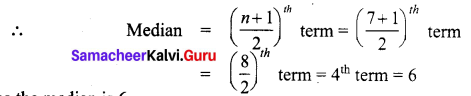Hence the median is 6.

Question 2.
Find the median: 11, 14, 10, 9, 14, 11, 12, 6, 7, 7.
Solution:
Arranging in ascending order: 6, 7, 7, 9, 10, 11, 11, 12, 14, 14
Here n = 10, which is even.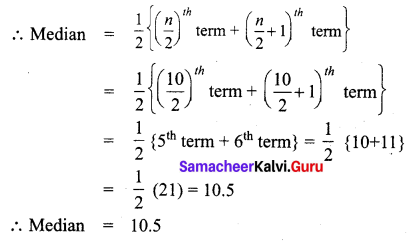Think (Text book Page No. 108)

Complete the table given below and observe it to answer the following questions.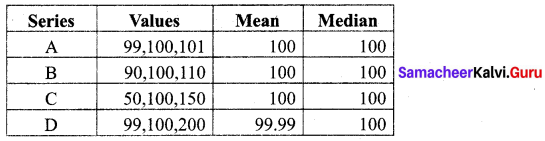Solution:

Question 1.
Which are all the series having common mean and median?
Solution:
Solution:
A, B and C.

Question 2.
Why median is same for all the 4 series?
Solution:
Since the middle value is 100.Question 3.
How mean is unchanged in the series A, B and C ?
Solution:
The difference between the given numbers are equal.

Question 4.
What change is to be made in the data, so that mean and median of ‘D’ series is equal to other series?
Solution:
If 99 becomes 0 or 200 becomes 101 then mean becomes 100.